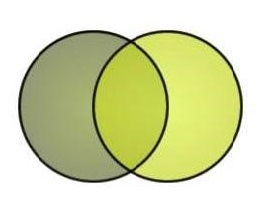# complex plane

n=3

a: complex plane ~
b: an ordinary x-y graph

What:

"The complex plane is like an ordinary x, y graph except that it contains both real and imaginary numbers--numbers containing a term called i, which represent the square root of -1. Real numbers are plotted on the x-axis imaginary numbers on the y axis."

Useful?
Writer: Jeff Prosise
LCC:
Where:
Date: Aug 21 2014 5:13 PM

a: Complex plane ~
b: the usual 2D xy plane

What: "The complex plane is like the usual 2D xy plane you're used to but instead of x and y axes we have the real and imaginary axes. You can then plot complex numbers geometric ally quite easily - by plotting the two parts of the number against their respective axes."

Useful?
Writer: Not Stated
LCC:
Where:
Date: Aug 21 2014 5:17 PM

a: Complex plane ~
b: the paper that the fractal image is drawn on

What: "The bottom line is that the complex plane is like the "paper" that the fractal image is drawn on. Actually, that reminds me of something-I don't think I've mentioned this yet, but the main reason that fractal images were not appreciated until quite recently is that they can only be drawn in a reasonable amount of time by using a computer to do the massive amount of computation necessary to render them."

Useful?
Writer: Not Stated
LCC: